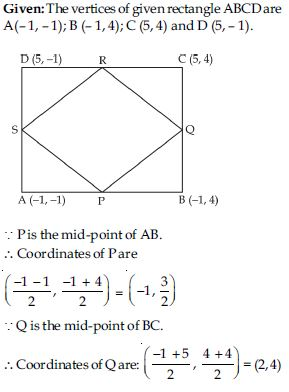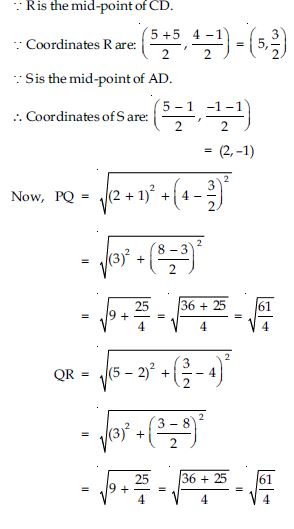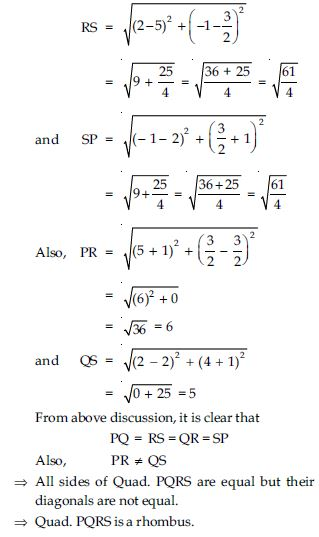# NCERT Solutions for Class 10 Math Chapter 7 - Coordinate Geometry

The educational resources including NCERT Solutions at Aasoka are designed as per the latest exam pattern and syllabus. This helps students in covering the entire syllabus so that they can get exam-ready. With the help of NCERT Solutions for Class 10th, students can regularly practice their mathematical skills. Regular practice will help students achieve good grades in their board exams.

“Coordinate Geometry” chapter of Class 10th teaches students to locate a given point with an ordered pair of numbers. With Cartesian or coordinate geometry, students can find the distance between the two points whose coordinates are provided. Along with this, the way to find the coordinates of the point which bisects a line segment joining two given points in a given ratio is described. The chapter will also explain about section formula, areas of triangles, and distance formula.

##### Question 1:

Find the distance between the following pairs of points: (2, 3), (4, 1)

Given points are: (2, 3), (4, 1)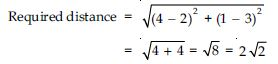##### Question 2:

Find the distance between the following pairs of points: (– 5, 7), (– 1, 3)

Given points are: (– 5, 7), (– 1, 3)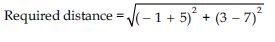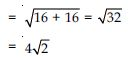##### Question 3:

Find the distance between the following pairs of points: (a, b), (– a, – b).

Given points are: (a, b), (– a, – b)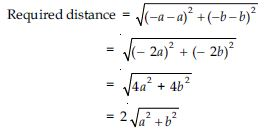##### Question 4:

Find the distance between the points (0, 0) and (36, 15). Can you now find the distance between the two towns A and B discussed in section 7.2.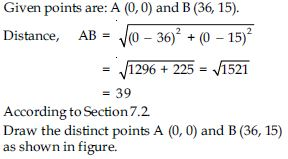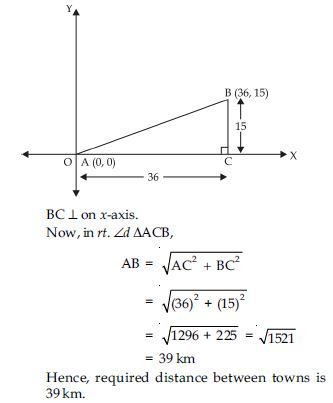##### Question 5:

Determine if the points (1, 5), (2, 3) and (– 2, – 11) are collinear.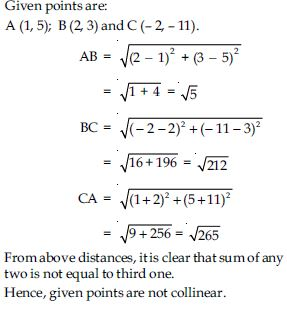##### Question 6:

Check whether (5, –2), (6, 4) and (7, –2) are the vertices of an isosceles triangle.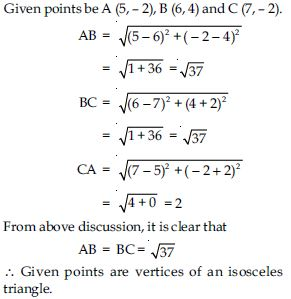##### Question 7:

In a classroom, 4 friends are seated at the points A, B, C and D as shown in fig. Champa and Chameli walk into the class and after observing for a few minutes Champa asks Chameli, ‘‘Don’t you think ABCD is a square’’ ? Chameli disagrees. Using distance formula, find which of them is correct, and why?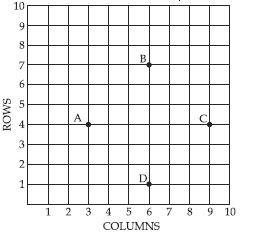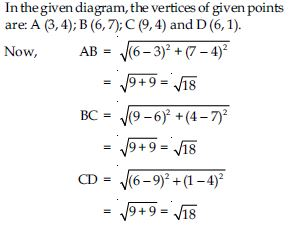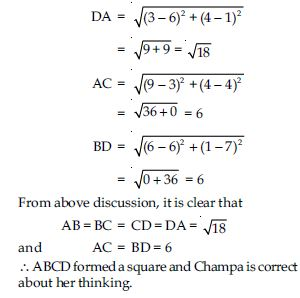##### Question 8:

Name the type of quadrilateral formed, if any, by the following points, and give reasons for your answer: (– 1, – 2), (1, 0), (– 1, 2), (– 3, 0)

Given points be A (– 1, – 2); B (1, 0); C (– 1, 2)
and D (– 3, 0).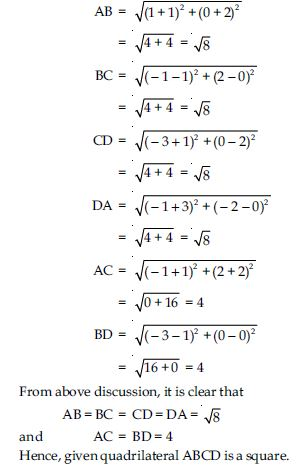##### Question 9:

Name the type of quadrilateral formed, if any, by the following points, and give reasons for your answer: (– 3, 5), (3, 1), (0, 3), (– 1, – 4)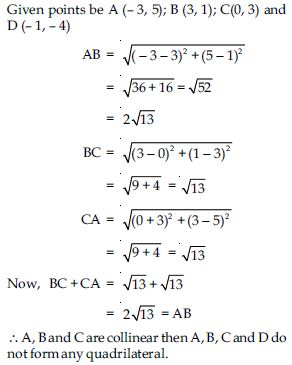##### Question 10:

Name the type of quadrilateral formed, if any, by the following points, and give reasons for your answer: (4, 5), (7, 6), (4, 3), (1, 2)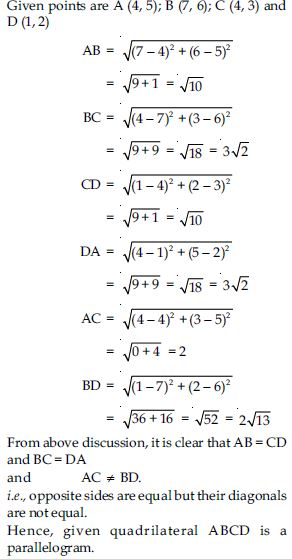##### Question 11:

Find the points on the x-axis which is
equidistant from (2, – 5) and (– 2, 9).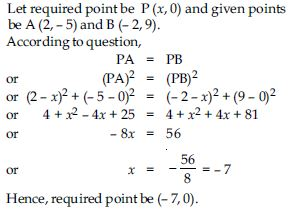##### Question 12:

Find the values of y for which the distance between the points P (2, – 3) and Q (10, y) is 10 units.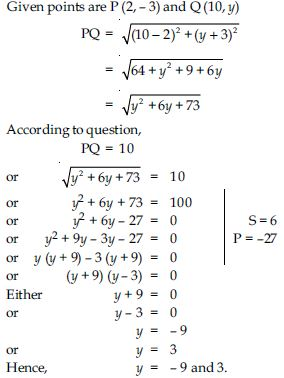##### Question 13:

If Q (0, 1) is equidistant from P (5, –3) and R (x, 6), find the values of x. Also find the distances QR and PR.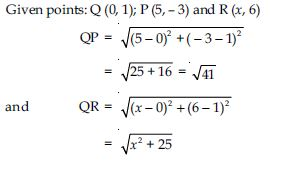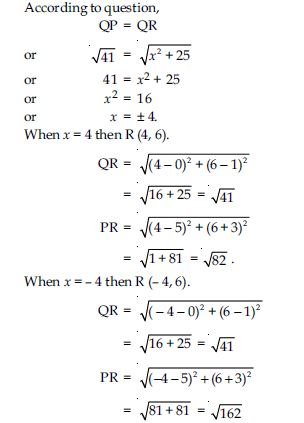##### Question 14:

Find a relation between x and y such that the points (x, y) is equidistant from the point (3, 6) and (–3, 4).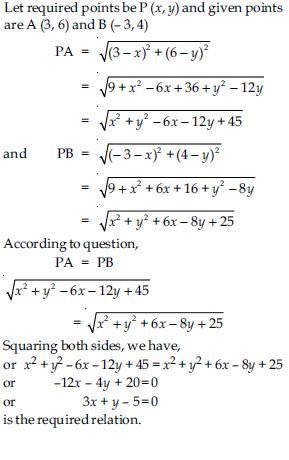##### Question 15:

Find the coordinates of the point which divides the join (–1, 7) and (4, – 3) in the ratio 2 : 3.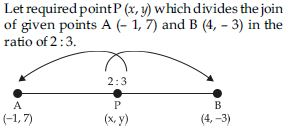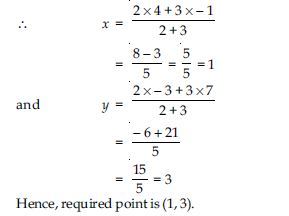##### Question 16:

Find the coordinates of the points of trisection of the line segment joining (4, – 1) and (–2, – 3).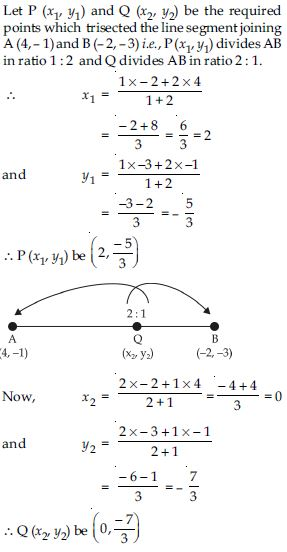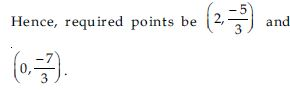##### Question 17: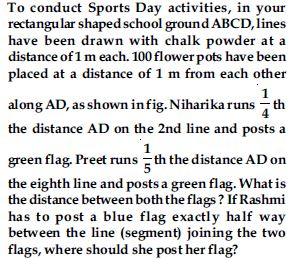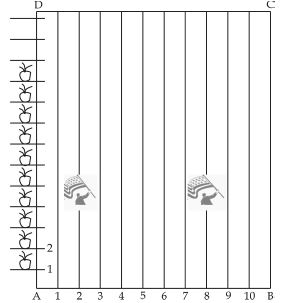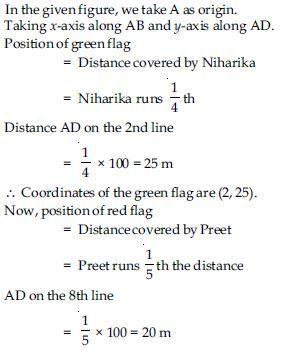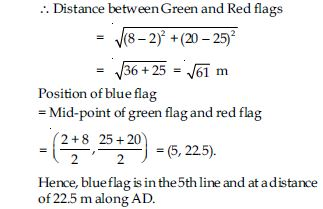##### Question 18:

Find the ratio in which the segment joining the points (– 3, 10) and (6, – 8) is divided by (– 1, 6).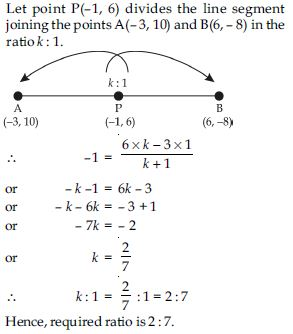##### Question 19:

Find the ratio in which the line segment joining A (1, – 5) and B (– 4, 5) is divided by the x-axis. Also find the coordinates of the point of division.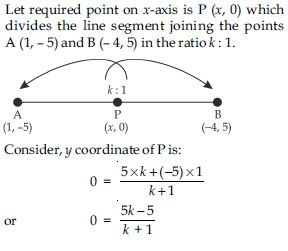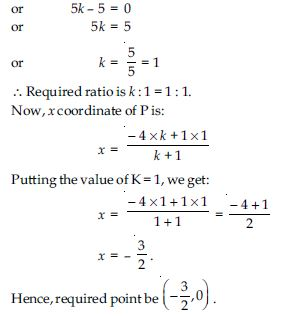##### Question 20:

If (1, 2) ; (4, y) ; (x, 6) and (3, 5) are the vertices of a parallelogram taken in order, find x and y.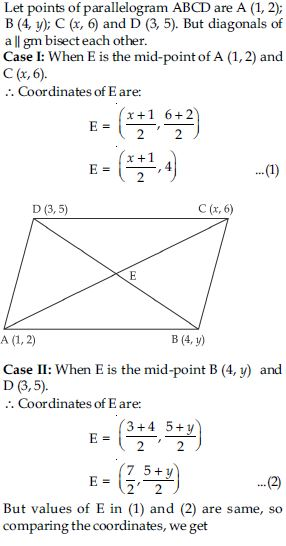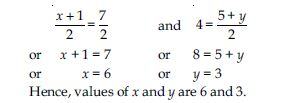##### Question 21:

Find the coordinates of a point A, where AB is the diameter of a circle whose centre is (2, – 3) and B is (1, 4).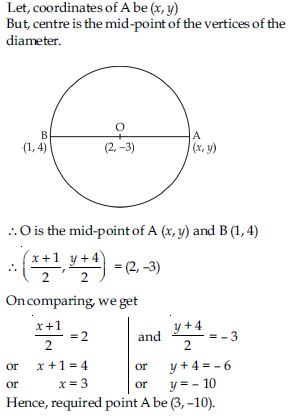##### Question 22: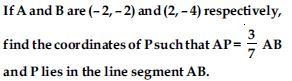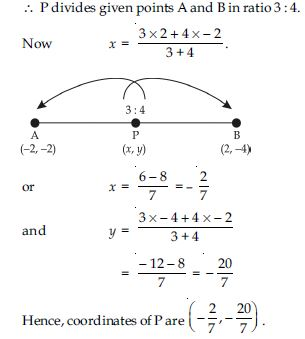##### Question 23:

Find the coordinates of the points which divide the line segment joining A (– 2, 2) and B (2, 8) into four equal parts.

Let required points are C, D and E which divide the line segment joining the points A (– 2, 2) and B (2, 8) into four equal parts. Then D is mid-point of A and B; C is the midpoint of A and D; E is the mid-point of D and B such that: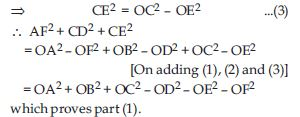##### Question 24:

Find the area of a rhombus if the vertices are (3, 0); (4, 5); (–1, 4) and (–2, –1) taken in order.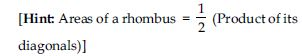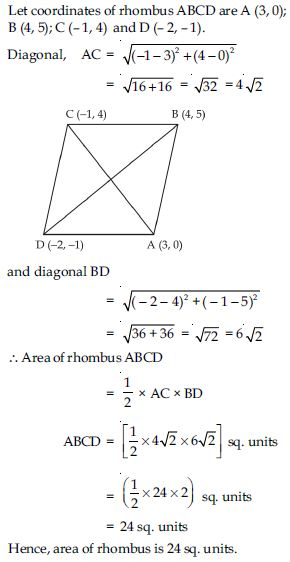= 24 sq. units Hence, area of rhombus is 24 sq. units.

##### Question 25:

Find the area of the triangle whose vertices are: (2, 3), (–1, 0), (2, – 4)

Let vertices of the $∆$ABC are A (2, 3), B (–1, 0) and C (2, – 4).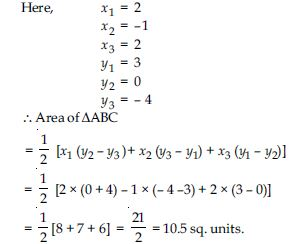##### Question 26:

Find the area of the triangle whose vertices are: (– 5, –1), (3, – 5), (5, 2)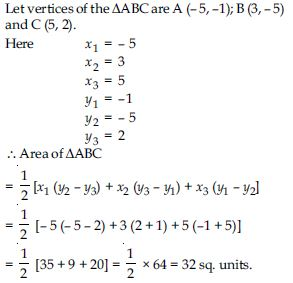##### Question 27:

In each of the following find the value of ‘k’ for which the points are collinear. (7, – 2), (5, 1), (3, k)

Let given points be: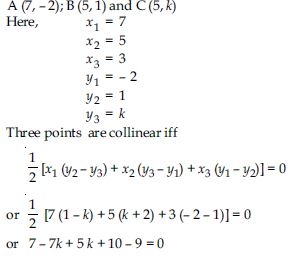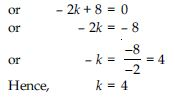##### Question 28:

In each of the following find the value of ‘k’ for which the points are collinear.

(8, 1), (k, – 4), (2, – 5)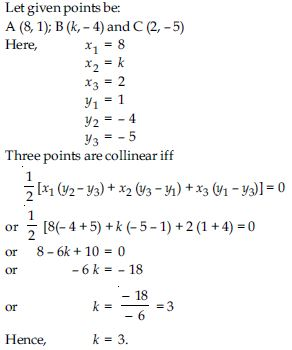##### Question 29:

Find the area of the triangle formed by joining the mid-points of the sides of the triangle whose vertices are (0, –1), (2, 1) and (0, 3). Find the ratio of the area of the triangle formed to the area of the given triangle.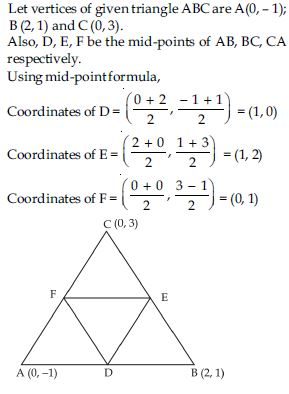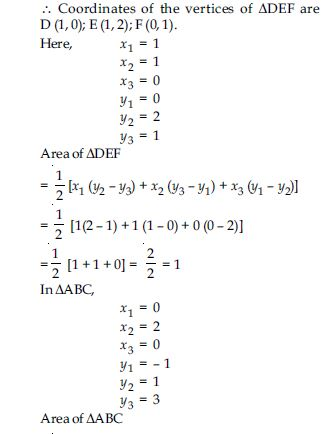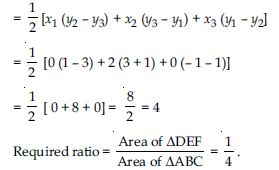##### Question 30:

Find the area of the quadrilateral whose
vertices taken in order, are (– 4, – 2), (– 3, –5), (3, – 2), (2, 3).

Let coordinates of the given quadrilateral ABCD
are A (– 4, – 2); B (– 3, – 5); C (3, – 2) and D (2, 3).
Join AC then Quad. ABCD divides in two
triangles i.e., $∆$ABC and $∆$CDA.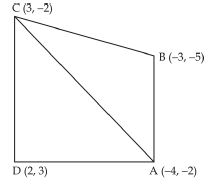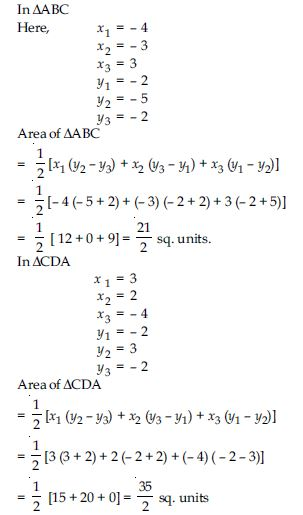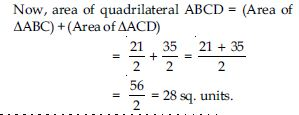##### Question 31:

You have studied in class IX (Ch. 9, Ex. 3)that a median of a triangle divides it into two triangles of equal areas. Verify this result for $∆$ABC whose vertices are A(4, – 6), B(3, – 2) and C(5, 2).

Given that coordinates of the vertices of $∆$ABC
are A(4, – 6); B (3, – 2) and C (5, 2).
Let CD is the median i.e., D is the mid-point of
AB which divides $∆$ABC into two parts i.e.,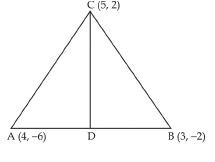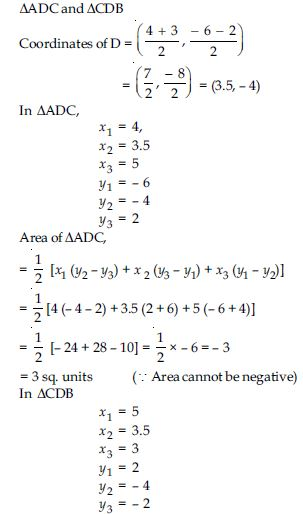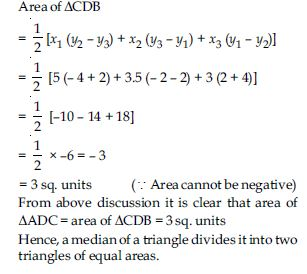##### Question 32:

Determine the ratio in which the line 2x + y – 4 = 0 divides the line segment joining the points A (2, – 2) and B (3, 7).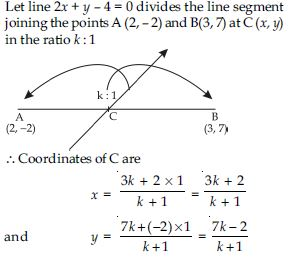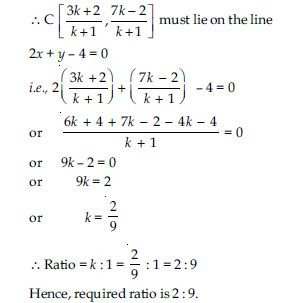##### Question 33:

Find a relation between x and y if the points
(x, y), (1, 2) and (7, 0) are collinear.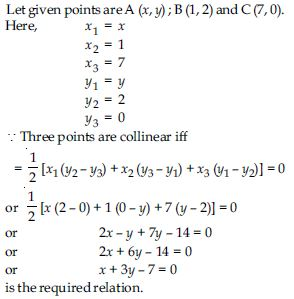##### Question 34:

Find the centre of a circle passing through the points (6, – 6); (3, – 7) and (3, 3).

Let O (x, y) be the required centre of the circle which passes through points P(6, –6);
Q (3, – 7) and R (3, 3).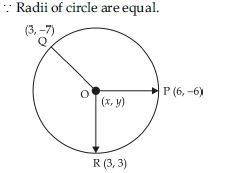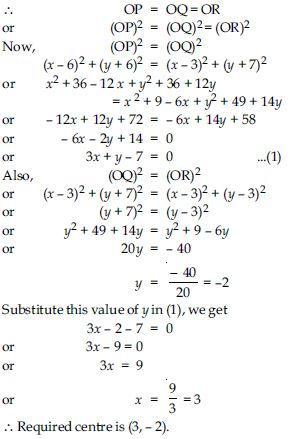##### Question 35:

The two opposite vertices of a square are (–1, 2) and (3, 2). Find the coordinates of other two vertices.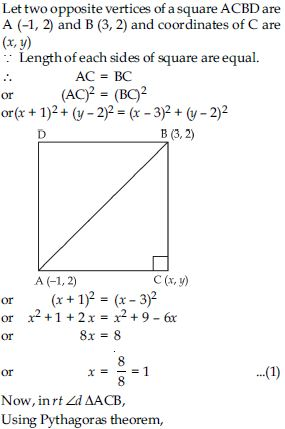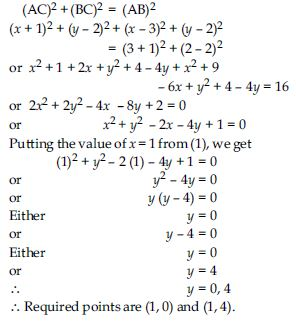##### Question 36:

The Class X students of a secondary school in Krishinagar have been allotted a rectangular plot of land for their gardening activity. Sapling of Gulmohar are planted on the boundary at a distance of 1 m from each other. There is a triangular grassy lawn in the plot as shown in the fig. The students are to sow seeds of flowering plants on the remaining area of the plot.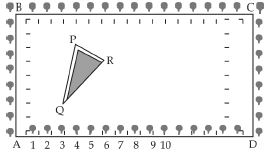(i) Taking A as origin, find the coordinates of the vertices of the triangle.
(ii) What will be the coordinates of the vertices of $∆$PQR if C is the origin? Also calculate the arc of the triangle in these cases. What do you observe?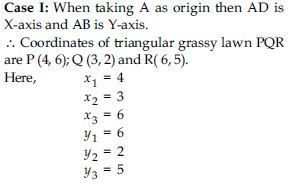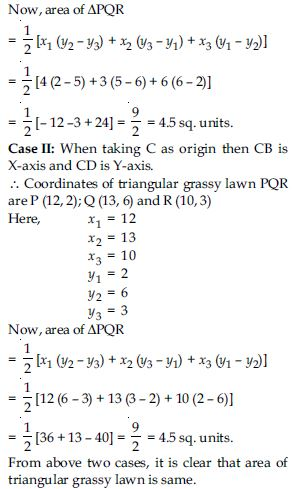##### Question 37: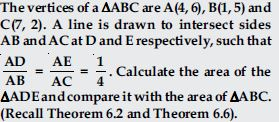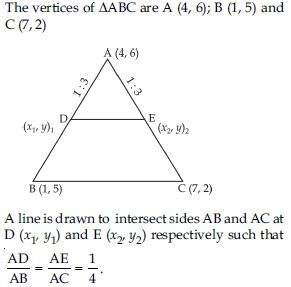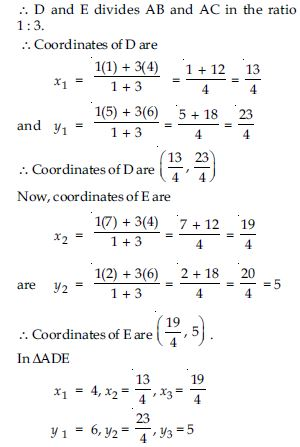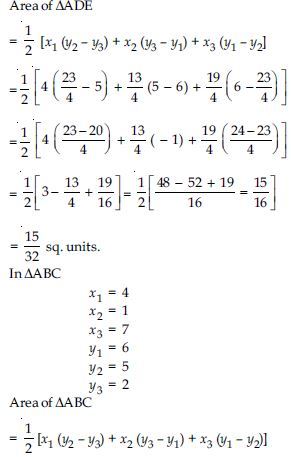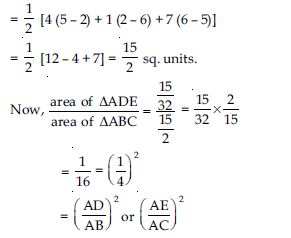##### Question 38:

Let A(4, 2), B (6, 5) and C (1, 4) be the vertices of $∆$ABC. The median from A meets BC at D. Find the coordinates of the point D.
[Note: The point which is common to all the three medians is called centroid and this point divides each median in the ratio 2 : 1.]

Given that vertices of $∆$ABC are A (4, 2); B (6, 5) and C (1, 4). AD is the median from the vertex A.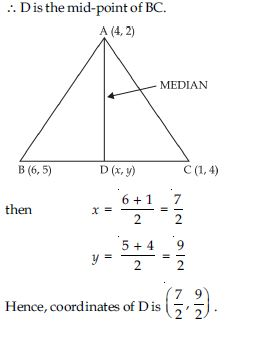##### Question 39:

Let A(4, 2), B (6, 5) and C (1, 4) be the vertices of $∆$ABC. Find the coordinates of the point P on AD such that AP : PD = 2 : 1.
[Note: The point which is common to all the three medians is called centroid and this point divides each median in the ratio 2 : 1.]

Given that vertices of $∆$ABC are A (4, 2); B (6, 5)
and C (1, 4).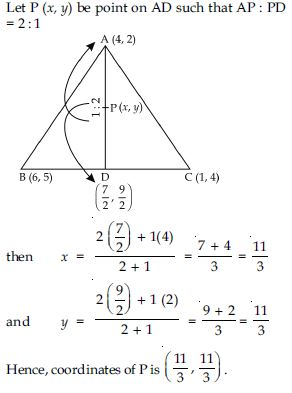##### Question 40:

Let A(4, 2), B (6, 5) and C (1, 4) be the vertices of $∆$ABC. Find the coordinates of points Q and R on medians BE and CF respectively such that BQ : QE = 2 : 1 and CR : RF = 2 : 1.
[Note: The point which is common to all the three medians is called centroid and this point divides each median in the ratio 2 : 1.]

Given that vertices of $∆$ABC are A (4, 2); B (6, 5)
and C (1, 4).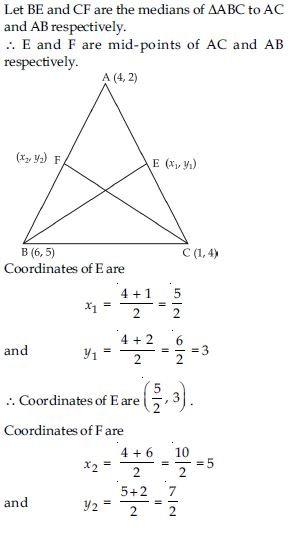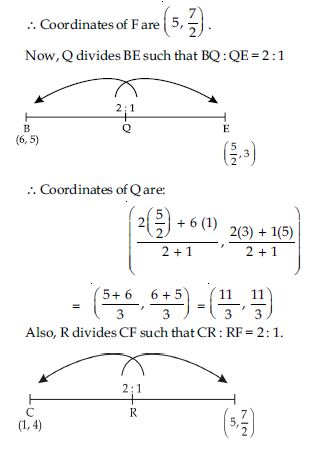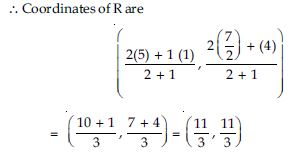##### Question 41: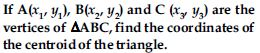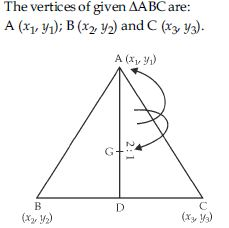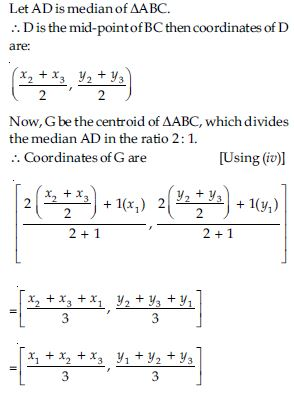##### Question 42:

ABCD is a rectangle formed by the points A(– 1, – 1), B (– 1, 4), C (5, 4) and D (5, – 1). P, Q, R and S are the mid-points of AB, BC, CD and DA respectively. Is the quadrilateral PQRS a square? a rectangle? or a rhombus? Justify your answer.